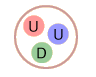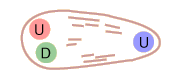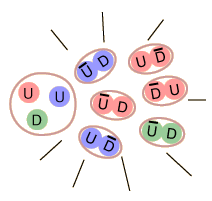# Bag Model of Quark Confinement

In dealing with the nature of quark confinement, one visualization is that of an elastic bag which allows the quarks to move freely around, as long as you don't try to pull them further apart. But if you try to pull a quark out, the bag stretches and resists.The quarks of a proton are free to move within the proton volume If you try to pull one of the quarks out, the energy required is on the order of 1 GeV per fermi, like stretching an elastic bag. The energy required to produce a separation far exceeds the pair production energy of a quark-antiquark pair, so instead of pulling out an isolated quark, you produce mesons as the produced quark-antiquark pairs combine.

The models of quark confinement help in understanding why we have not seen isolated quarks. If one of the constituent quarks of a particle is given enough energy, it can create a jet of mesons as the energy imparted to the quark is used to produce quark-antiquark pairs.

Experiments show that the forces containing the quarks get weaker as the quarks get closer together, so that within the confines of a baryon or hadron, they are essentially free to move about. This condition is referred to as "asymptotic freedom".

Index

Particle concepts

Reference
Rohlf
Ch. 18

 HyperPhysics***** Quantum Physics R Nave
Go Back

# Asymptotic Freedom

As the quarks within a meson or baryon get closer together, the force of containment gets weaker so that it asymptotically approaches zero for close confinement. The implication is that the quarks in close confinement are completely free to move about. Part of the nature of quark confinement is that the further you try to force the quarks apart, the greater the force of containment. This is often visualized in terms of the "bag model" of quark confinement.

A potential function which has been successfully used to describe some quark systems is of the form:The quark-quark coupling strength decreases for small values of r, and Rohlf describes this qualitatively as resulting from the penetration of the gluon cloud surrounding the quarks. The gluons carry "color charge" and therefore the penetration of the cloud would reduce the effective color charge of the quark.

Another approach to asymptotic freedom is to use a variable strong force coupling constant which depends upon the wavelength of the quark. An expression which comes from quantum chromodynamics is:The nature of this relationship is that it gives a value of about 1 for the coupling constant at the radius of a proton, and this is the value conventionally used to describe the strength of the strong interaction within nuclei. When the proton is penetrated to a radius corresponding to an energy of 1 TeV, the coupling constant is down to about 0.1, another manifestation of asymptotic freedom.

Index

Particle concepts

Reference
Rohlf
Ch. 17,18

 HyperPhysics***** Quantum Physics R Nave
Go Back